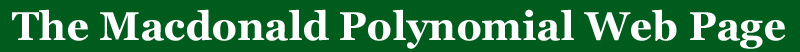BACK TO:# The amazing Operator(nabla)

Define an operator acting on symmetric functions that has the familyas eigenfunctions as= tn(µ)qn(µ')where n(µ) is the sum of µi(i-1).

These are some of the amazing conjectures associated with:

Conjecture 1en(X) is the Frobenius series for the  space of diagonal harmonics

If the coefficient of en(X) is taken in this symmetric function, we have the definition of the q,t-Catalan numbers as they are defined in this web site.
If the coefficient of e1n(X) is taken in this symmetric function, we have (conjectured) the Hilbert series for the space of diagonal harmonics and the dimension of this space is conjectured to be (n+1)n-1, the number of rooted forests on n labeled verticies.

Conjecture 2sµ(X) is totally Schur positive or totally Schur negative (a formula for the sign of this symmetric function is also conjectured)

It is not obvious from the definition that the operator nabla gives polynomial coefficients when it acts on the Schur symmetric functions.  The proof of this theorem was the first proof that the q,t-Catalan numbers were polynomials in q and t.  A resolution of either of the conjectures above would also imply that the q,t-Catalan numbers are polynomials with non-negative integer coefficients.

Conjecture 3mµ(X) is totally Schur positive or totally Schur negative

Conjecture 4Hµ(X;1/t) is totally Schur positive or totally Schur negative

Conjecture 5Hµ(X;t) is Schur positive

It seems that <H(k1(n-k))(X;t), h1n> is a q,t analog of the numbers (k+1)k-1 (n)(n-k)

For some more of the amazing properties of this operator see the references below:

Maple functions for this operator are available on this web site if you are interested in exploring further.  These functions require the package SF.